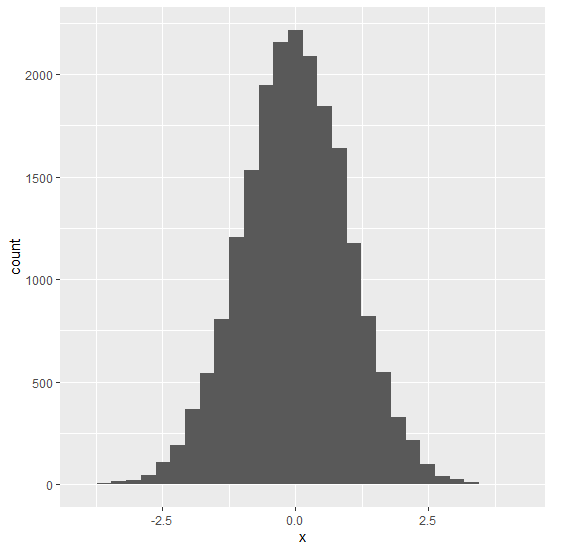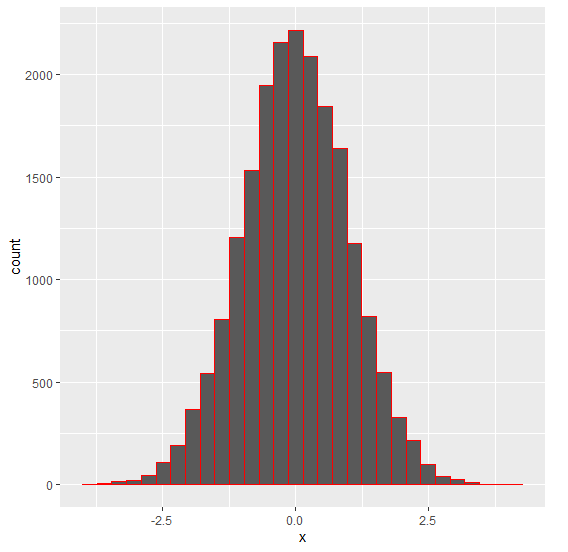# Change the outline color for histogram bars using ggplot2 in R.

To change the outlines color of histogram bars using ggplot2, we can use col argument inside geom_histogram function of ggplot2 package.

For Example, if we have a data frame called df that contains a column say X then we can create the histogram of X with different outline color of bars using the below command −

ggplot(df,aes(X))+geom_histogram(bins=30,col=I("red"))

## Example

Following snippet creates a sample data frame −

x<-rnorm(20000)
df<-data.frame(x)
head(df,20)

The following dataframe is created −

          x
1 -1.31426410
2 -2.62316895
3 -0.19231545
4  0.89701476
5 -0.10409584
6  0.23481007
7  1.53117888
8 -0.63855632
9  0.85309492
10  0.04791316
11  0.37854603
12  1.24928336
13 -0.65170883
14  2.58938742
15 -0.82602063
16  0.97524621
17  0.36930032
18  0.07720108
19 -0.66050878
20  0.66199644

To load the ggplot2 package and to create a histogram of x, on the above created data frame, add the following code to the above snippet −

x<-rnorm(20000)

library(ggplot2)
ggplot(df,aes(x))+geom_histogram(bins=30)

## Output

If you execute all the above given snippets as a single program, it generates the following Output −To create a histogram of x with red colored outline of bars, on the above created data frame, add the following code to the above snippet −

x<-rnorm(20000)

library(ggplot2)
ggplot(df,aes(x))+geom_histogram(bins=30,col=I("red"))

## Output

If you execute all the above given snippets as a single program, it generates the following Output −